# Dear Family, your Operation Maths guide to Directed Numbers

## Dear Family, your Operation Maths guide to Directed Numbers

Category : Uncategorized

Dear Family, below is a brief guide to understanding the topic of directed numbers (fifth and sixth classes only), as well as some practical suggestions as to how you might support your children’s understanding at home. Also below, are a series of links to digital resources that will help both the children, and you, learn more about directed numbers.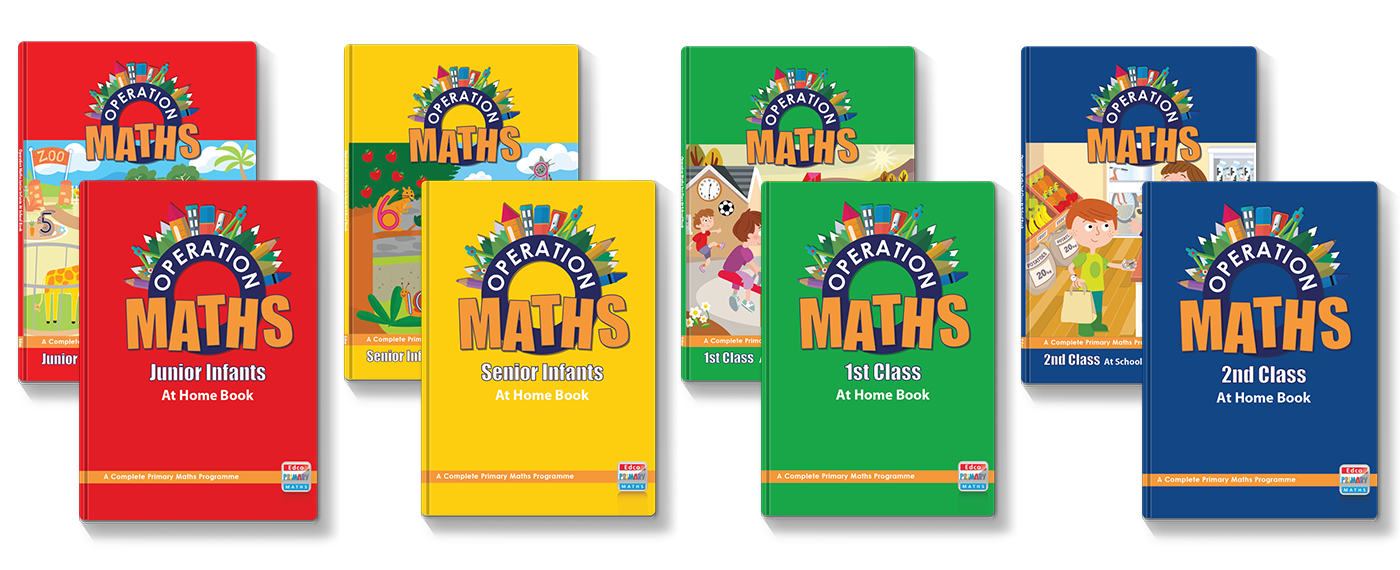You can also find class specific tips in the Operation Maths Dear Family letters for fifth and sixth class.

#### Understanding Directed Numbers

Directed Numbers are numbers with both size and direction; one direction is positive, and the other is negative. For example, temperature is typically described in a number of degrees either above zero (positive values) or below zero (negative values). Positive and negative numbers are also referred to as integers.

In Operation Maths, the children are first introduced to directed numbers in fifth class, where the focus is on the children appreciating where directed numbers can be encountered in real life, for example:

• Temperature
• Bank statements/ money accounts: having money is shown as positive (+) and owing money or overdrafts are shown as negative (-)
• Elevations above and below sea level
• Floors below the ground floor in a large building are often labeled as -1, -2 etc
• Golf scores are written as above and below par
• Goal difference in soccer league tables
• Depths in a swimming pool

In school, the children are encouraged to use the words positive and negative, rather than plus and minus; for example for the value -6 we should say negative six rather than minus six. This is particularly important for when the children start adding positive and negative numbers (in Operation Maths 6): for example (–3) + (+9) should be read as ‘negative three add/plus positive nine’ rather than ‘minus 3 plus plus 9’. It is also important that the children recognise that positive numbers can be written either with, or without, the positive sign, therefore we can assume that any number, without a sign, is positive.

#### Practical Suggestions for all Children

• Draw your child’s attention to wherever they or you encounter directed numbers (see above for possible examples).
• If your child is having difficulty visualising, comparing, ordering etc directed numbers, encourage them to think of a real example. One of the most-relatable of these is that of temperatures and the thermometer. Look at a real thermometer or use an online virtual example such as this one.
• As explained above, encourage your child to use the words positive and negative, rather than plus and minus, when describing directed numbers.

#### Digital Resources for Fifth and Sixth ClassesIntegers: Video lesson that introduces integers (positive and negative numbers) and where they occur in real lifeMashup Math – Elevation above or below sea-level: A video lesson that explores elevation as examples of positive and negative numbers.Negative Numbers: A series of video lessons from White Rose Maths, including Introducing Negative Numbers (Year 4), Negative Numbers (Year 4), Negative Numbers (Year 5), Negative Numbers in Context (Year 6), Negative Numbers (Year 6), Add and Subtract Integers (Year 6).Khan Academy Negative Numbers: A series of videos and practice questions exploring negative numbers. Afterwards, for more of a challenge, look at Integers and Whole Numbers. You can also register for a free Khan Academy account to record your progress and explore other topics/grades.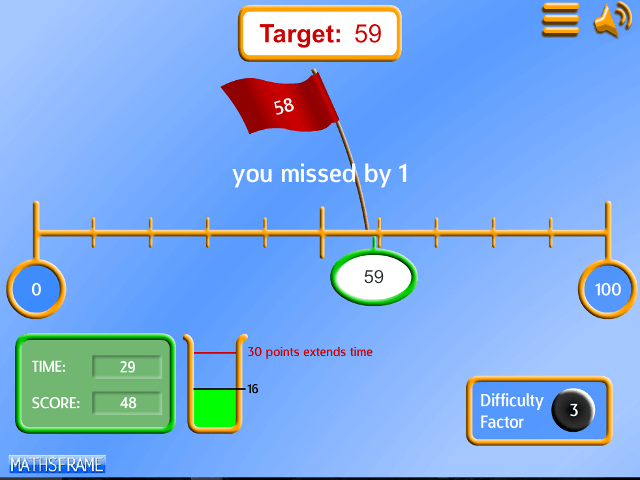Maths Frame – Empty Number Line: Practice placing positive and negative integers in the correct position; choose -5 to 5 initially and then move to the next levels to challenge yourself.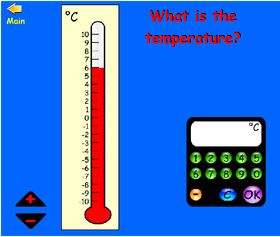What is the Temperature? Identify the temperature shown on the thermometers. A way to practice directed numbers in a real-life context. Includes values above and below zero, and has options for various ranges.Caterpillar Ordering: Choose Ordering and then -10 to 10 to order integers.Coconut Ordering: Hit the numbers in order of size. Select ‘numbers’ and then choose from numbers from -10 to 10 to order integers.Number Lines in Disguise: A challenge and interactive game (scroll down) from NRICH; Can you work out the number marked by the dot? Includes positive and negative numbers.That Quiz: Inequalities for comparing numbers, Arithmetic for addition calculations involving directed numbers and Number Line for identifying numbers by their position. In each of these activities there is the option to include negative numbers along with positive; just make sure the the “negative” option is selected on the left hand side.Number Line App: This virtual tool can be used to explore the position of values on a number line,including positive and negative numbers; also to model addition and subtraction involving positive and negative numbers.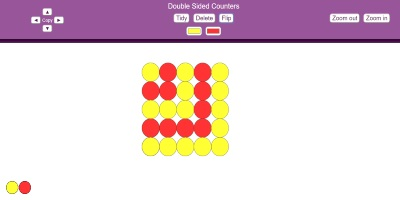Directed Numbers Counters: These double sided counters can be used to model addition and subtraction involving positive and negative numbers (ensure that the “sign” option is ticked).Integers (Directed Numbers): a selection of practice games from ixl.com. You can do a number of free quizzes each day without having a subscription. Start with the second class games and work up through the activities.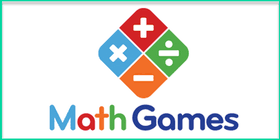Integers: Practice games from Math Games. Choose your class level.